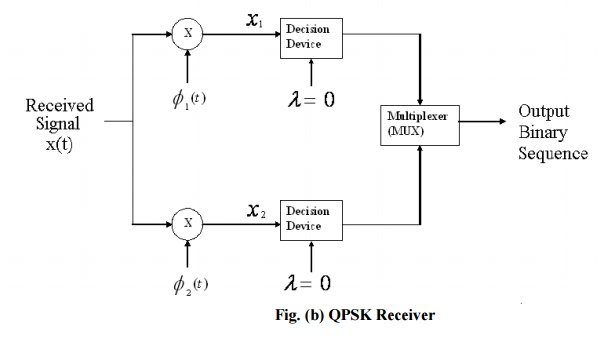Home | | Digital Communication | Quadrature Phase Shift Keying (QPSK)

# Quadrature Phase Shift Keying (QPSK)

In a sense, QPSK is an expanded version from binary PSK where in a symbol consists of two bits and two orthonormal basis functions are used.

QUADRATURE PHASE - SHIFT KEYING (QPSK)

In a sense, QPSK is an expanded version from binary PSK where in a symbol consists of two bits and two orthonormal basis functions are used. A group of two bits is often called a “dibit”. So, four dibits are possible. Each symbol carries same energy. Let, E: Energy per Symbol and T: Symbol duration = 2.* Tb, where Tb: duration of 1 bit.In QPSK system the information carried by the transmitted signal is contained in the phase.

The QPSK receiver consists of a pair of correlators with a common input and supplied with a locally generated pair of coherent reference signals ᶲ1(t) &ᶲ2(t)as shown in fig(b).The correlator outputs x1 and x2 produced in response to the received signal x(t) are each compared with a threshold value of zero.

## The in-phase channel output:

If x1> 0 a decision is made in favour of symbol 1 x1< 0 a decision is made in favour of symbol 0.

If x2>0 a decision is made in favour of symbol 1 and x2<0 a decision is made in favour of symbol 0 Finally these two binary sequences at the in phase and quadrature channel outputs are combined in a multiplexer (Parallel to Serial) to reproduce the original binary sequence## Probability of error:-

A QPSK system is in fact equivalent to two coherent binary PSK systems working in parallel and using carriers that are in-phase and quadrature. The in-phase channel output x1 and the Q-channel output x2 may be viewed as the individual outputs of the two coherent binary PSK systems. Thus the two binary PSK systems may be characterized as follows.

-  The signal energy per bit  √E/2

-  The noise spectral density is N0/2

The bit errors in the I-channel and Q-channel of the QPSK system are statistically independent .The I-channel makes a decision on one of the two bits constituting a symbol (di bit) of the QPSK signal and the Q-channel takes care of the other bit.

Study Material, Lecturing Notes, Assignment, Reference, Wiki description explanation, brief detail
Digital Communication : Digital Modulation Scheme : Quadrature Phase Shift Keying (QPSK) |